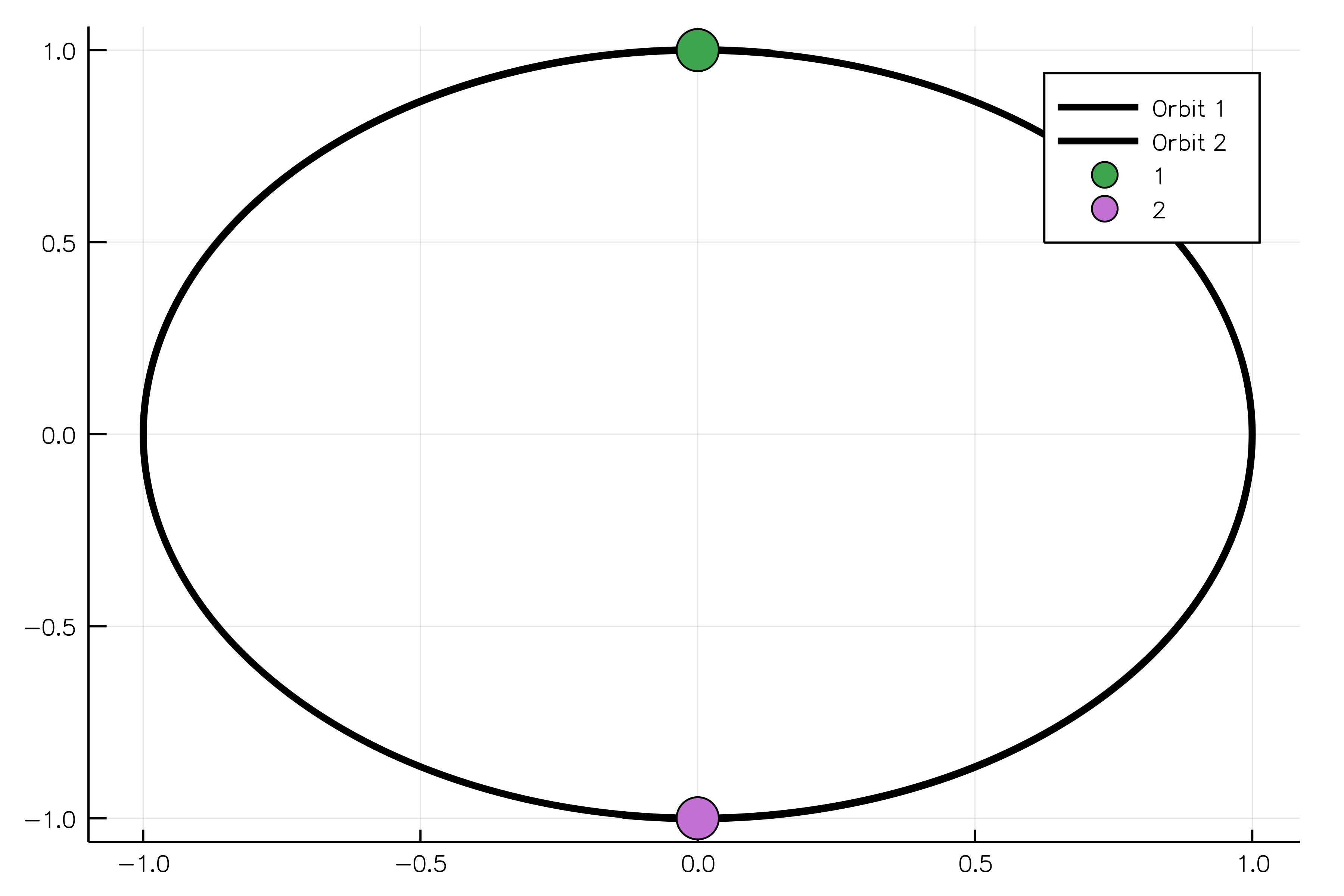## DiffEqPhysics.jl

A library for building differential equations arising from physical problems
Author SciML
Popularity
28 Stars
Updated Last
12 Months Ago
Started In
April 2017

# DiffEqPhysics

## Simulation of gravitationally interacting bodies

In order to create bodies/particles for the problem, one needs to use the MassBody structure and its constructor, which accepts mass, initial coordinates and velocity of the body.

```body1 = MassBody(2.0, SVector(0.0, 1.0, 0.0), SVector( 5.775e-6, 0.0, 0.0))
body2 = MassBody(2.0, SVector(0.0,-1.0, 0.0), SVector(-5.775e-6, 0.0, 0.0))```

Usually we solve an n-body problem for a certain period of time:

`tspan = (0.0, 1111150.0);`

Solving gravitational problem one needs to specify the gravitational constant G.

`G = 6.673e-11`

In fact, now we have enough parameters to create an NBodyGravProblem object:

`problem = NBodyGravProblem([body1,body2], G, tspan)`

Solution to the problem might be evaluated using the standard `solve` function:

`solution = solve(problem, Tsit5());`

And, finally, we plot our solution showing two equal bodies rotating on the same orbit:

`plot_xy_scattering(solution,"./anim_two_boddies_scattering.gif")`### Required Packages

View all packages